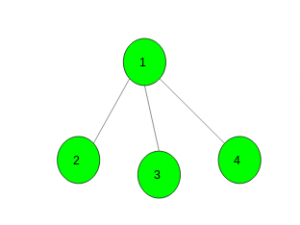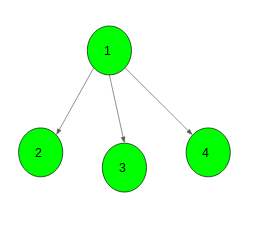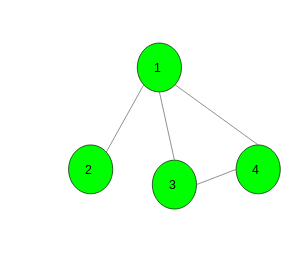# Convert the undirected graph into directed graph such that there is no path of length greater than 1

Given an undirected graph with N vertices and M edges and no self loops or multiple edges. The task is to convert the given undirected graph into a directed graph such that there is no path of length greater than 1. If it is possible to make such a graph then print two space-separated integers u and v in M lines where u, v denotes source and destination vertices respectively. If not possible then print -1.

Examples:

Input:Output:
1 2
1 3
1 4Input:Output: -1
For the given graph it is not possible to get a directed graph
such that there is no path of length greater than 1

## Recommended: Please try your approach on {IDE} first, before moving on to the solution.

Approach: Let suppose the graph contains a cycle of odd length. It means that some two consecutive edges of this cycle will be oriented in the same way and will form a path of length two. Then the answer is -1.

And if the graph contains no cycles of odd length. Then it is bipartite. Let’s color it and see what we got. We got some vertices in the left part, some vertices in the right part and all edges connecting vertices from different parts. Let’s orient all edges such that they will go from the left part to the right part.

Below is the implementation of the above approach:

## C++

 `// C++ implementation of the approach ` `#include ` `using` `namespace` `std; ` `#define N 100005 ` ` `  `// To store the graph ` `vector<``int``> gr[N]; ` ` `  `// To store colour of each vertex ` `int` `colour[N]; ` ` `  `// To store edges ` `vector > edges; ` ` `  `// To check graph is bipartite or not ` `bool` `bip; ` ` `  `// Function to add edges ` `void` `add_edge(``int` `x, ``int` `y) ` `{ ` `    ``gr[x].push_back(y); ` `    ``gr[y].push_back(x); ` `    ``edges.push_back(make_pair(x, y)); ` `} ` ` `  `// Function to check given graph ` `// is bipartite or not ` `void` `dfs(``int` `x, ``int` `col) ` `{ ` `    ``// colour the vertex x ` `    ``colour[x] = col; ` ` `  `    ``// For all it's child vertices ` `    ``for` `(``auto` `i : gr[x]) { ` `        ``// If still not visited ` `        ``if` `(colour[i] == -1) ` `            ``dfs(i, col ^ 1); ` ` `  `        ``// If visited and having ` `        ``// same colour as parent ` `        ``else` `if` `(colour[i] == col) ` `            ``bip = ``false``; ` `    ``} ` `} ` ` `  `// Function to convert the undirected ` `// graph into the directed graph such that ` `// there is no path of length greater than 1 ` `void` `Directed_Graph(``int` `n, ``int` `m) ` `{ ` ` `  `    ``// Initially each vertex has no colour ` `    ``memset``(colour, -1, ``sizeof` `colour); ` ` `  `    ``// Suppose bipartite is possible ` `    ``bip = ``true``; ` ` `  `    ``// Call bipartite function ` `    ``dfs(1, 1); ` ` `  `    ``// If bipartite is not possible ` `    ``if` `(!bip) { ` `        ``cout << -1; ` `        ``return``; ` `    ``} ` ` `  `    ``// If bipartite is possible ` `    ``for` `(``int` `i = 0; i < m; i++) { ` ` `  `        ``// Make an edge from vertex having ` `        ``// colour 1 to colour 0 ` `        ``if` `(colour[edges[i].first] == 0) ` `            ``swap(edges[i].first, edges[i].second); ` ` `  `        ``cout << edges[i].first << ``" "` `             ``<< edges[i].second << endl; ` `    ``} ` `} ` ` `  `// Driver code ` `int` `main() ` `{ ` `    ``int` `n = 4, m = 3; ` ` `  `    ``// Add edges ` `    ``add_edge(1, 2); ` `    ``add_edge(1, 3); ` `    ``add_edge(1, 4); ` ` `  `    ``// Function call ` `    ``Directed_Graph(n, m); ` ` `  `    ``return` `0; ` `} `

## Java

 `// Java implementation of the approach ` `import` `java.util.*; ` ` `  `class` `GFG ` `{ ` `static` `class` `pair ` `{  ` `    ``int` `first, second;  ` `    ``public` `pair(``int` `first, ``int` `second)  ` `    ``{  ` `        ``this``.first = first;  ` `        ``this``.second = second;  ` `    ``}  ` `}  ` ` `  `static` `int` `N = ``100005``; ` ` `  `// To store the graph ` `static` `Vector []gr = ``new` `Vector[N]; ` ` `  `// To store colour of each vertex ` `static` `int` `[]colour = ``new` `int``[N]; ` ` `  `// To store edges ` `static` `Vector edges = ``new` `Vector<>(); ` ` `  `// To check graph is bipartite or not ` `static` `boolean` `bip; ` ` `  `// Function to add edges ` `static` `void` `add_edge(``int` `x, ``int` `y) ` `{ ` `    ``gr[x].add(y); ` `    ``gr[y].add(x); ` `    ``edges.add(``new` `pair(x, y)); ` `} ` ` `  `// Function to check given graph ` `// is bipartite or not ` `static` `void` `dfs(``int` `x, ``int` `col) ` `{ ` `    ``// colour the vertex x ` `    ``colour[x] = col; ` ` `  `    ``// For all it's child vertices ` `    ``for` `(Integer i : gr[x]) ` `    ``{ ` `        ``// If still not visited ` `        ``if` `(colour[i] == -``1``) ` `            ``dfs(i, col ^ ``1``); ` ` `  `        ``// If visited and having ` `        ``// same colour as parent ` `        ``else` `if` `(colour[i] == col) ` `            ``bip = ``false``; ` `    ``} ` `} ` ` `  `// Function to convert the undirected ` `// graph into the directed graph such that ` `// there is no path of length greater than 1 ` `static` `void` `Directed_Graph(``int` `n, ``int` `m) ` `{ ` ` `  `    ``// Initially each vertex has no colour ` `    ``for` `(``int` `i = ``0``; i < N; i++) ` `        ``colour[i] = -``1``; ` ` `  `    ``// Suppose bipartite is possible ` `    ``bip = ``true``; ` ` `  `    ``// Call bipartite function ` `    ``dfs(``1``, ``1``); ` ` `  `    ``// If bipartite is not possible ` `    ``if` `(!bip)  ` `    ``{ ` `        ``System.out.print(-``1``); ` `        ``return``; ` `    ``} ` ` `  `    ``// If bipartite is possible ` `    ``for` `(``int` `i = ``0``; i < m; i++)  ` `    ``{ ` ` `  `        ``// Make an edge from vertex having ` `        ``// colour 1 to colour 0 ` `        ``if` `(colour[edges.get(i).first] == ``0``) ` `        ``{ ` `            ``Collections.swap(edges, edges.get(i).first,  ` `                                    ``edges.get(i).second); ` `        ``} ` ` `  `        ``System.out.println(edges.get(i).first + ``" "` `+  ` `                           ``edges.get(i).second); ` `    ``} ` `} ` ` `  `// Driver code ` `public` `static` `void` `main(String[] args) ` `{ ` `    ``int` `n = ``4``, m = ``3``; ` `    ``for` `(``int` `i = ``0``; i < N; i++) ` `        ``gr[i] = ``new` `Vector<>(); ` `         `  `    ``// Add edges ` `    ``add_edge(``1``, ``2``); ` `    ``add_edge(``1``, ``3``); ` `    ``add_edge(``1``, ``4``); ` ` `  `    ``// Function call ` `    ``Directed_Graph(n, m); ` `} ` `} ` ` `  `// This code is contributed by PrinciRaj1992 `

## Python3

 `# Python3 implementation of the approach ` ` `  `N ``=` `100005` `  `  `# To store the graph ` `gr ``=` `[[] ``for` `i ``in` `range``(N)] ` `  `  `# To store colour of each vertex ` `colour ``=` `[``-``1``] ``*` `N ` `  `  `# To store edges ` `edges ``=` `[] ` `  `  `# To check graph is bipartite or not ` `bip ``=` `True` `  `  `# Function to add edges ` `def` `add_edge(x, y): ` ` `  `    ``gr[x].append(y) ` `    ``gr[y].append(x) ` `    ``edges.append((x, y)) ` ` `  `# Function to check given graph ` `# is bipartite or not ` `def` `dfs(x, col): ` ` `  `    ``# colour the vertex x ` `    ``colour[x] ``=` `col ` `    ``global` `bip ` `  `  `    ``# For all it's child vertices ` `    ``for` `i ``in` `gr[x]:  ` `        ``# If still not visited ` `        ``if` `colour[i] ``=``=` `-``1``: ` `            ``dfs(i, col ^ ``1``) ` `  `  `        ``# If visited and having ` `        ``# same colour as parent ` `        ``elif` `colour[i] ``=``=` `col: ` `            ``bip ``=` `False` `     `  `# Function to convert the undirected ` `# graph into the directed graph such that ` `# there is no path of length greater than 1 ` `def` `Directed_Graph(n, m): ` ` `  `    ``# Call bipartite function ` `    ``dfs(``1``, ``1``) ` `  `  `    ``# If bipartite is not possible ` `    ``if` `not` `bip: ` `        ``print``(``-``1``) ` `        ``return` `     `  `    ``# If bipartite is possible ` `    ``for` `i ``in` `range``(``0``, m):  ` `  `  `        ``# Make an edge from vertex ` `        ``# having colour 1 to colour 0 ` `        ``if` `colour[edges[i][``0``]] ``=``=` `0``: ` `            ``edges[i][``0``], edges[i][``1``] ``=` `edges[i][``1``], edges[i][``0``] ` `  `  `        ``print``(edges[i][``0``], edges[i][``1``]) ` `  `  `# Driver code ` `if` `__name__ ``=``=` `"__main__"``: ` ` `  `    ``n, m ``=` `4``, ``3` `  `  `    ``# Add edges ` `    ``add_edge(``1``, ``2``) ` `    ``add_edge(``1``, ``3``) ` `    ``add_edge(``1``, ``4``) ` `  `  `    ``# Function call ` `    ``Directed_Graph(n, m) ` `  `  `# This code is contributed by Rituraj Jain `

## C#

 `// C# implementation of the approach ` `using` `System; ` `using` `System.Collections.Generic; ` `     `  `class` `GFG ` `{ ` ` `  `class` `pair ` `{  ` `    ``public` `int` `first, second;  ` `    ``public` `pair(``int` `first, ``int` `second)  ` `    ``{  ` `        ``this``.first = first;  ` `        ``this``.second = second;  ` `    ``}  ` `}  ` ` `  `static` `int` `N = 100005; ` ` `  `// To store the graph ` `static` `List<``int``> []gr = ``new` `List<``int``>[N]; ` ` `  `// To store colour of each vertex ` `static` `int` `[]colour = ``new` `int``[N]; ` ` `  `// To store edges ` `static` `List edges = ``new` `List(); ` ` `  `// To check graph is bipartite or not ` `static` `Boolean bip; ` ` `  `// Function to add edges ` `static` `void` `add_edge(``int` `x, ``int` `y) ` `{ ` `    ``gr[x].Add(y); ` `    ``gr[y].Add(x); ` `    ``edges.Add(``new` `pair(x, y)); ` `} ` ` `  `// Function to check given graph ` `// is bipartite or not ` `static` `void` `dfs(``int` `x, ``int` `col) ` `{ ` `    ``// colour the vertex x ` `    ``colour[x] = col; ` ` `  `    ``// For all it's child vertices ` `    ``foreach` `(``int` `i ``in` `gr[x]) ` `    ``{ ` `        ``// If still not visited ` `        ``if` `(colour[i] == -1) ` `            ``dfs(i, col ^ 1); ` ` `  `        ``// If visited and having ` `        ``// same colour as parent ` `        ``else` `if` `(colour[i] == col) ` `            ``bip = ``false``; ` `    ``} ` `} ` ` `  `// Function to convert the undirected ` `// graph into the directed graph such that ` `// there is no path of length greater than 1 ` `static` `void` `Directed_Graph(``int` `n, ``int` `m) ` `{ ` ` `  `    ``// Initially each vertex has no colour ` `    ``for` `(``int` `i = 0; i < N; i++) ` `        ``colour[i] = -1; ` ` `  `    ``// Suppose bipartite is possible ` `    ``bip = ``true``; ` ` `  `    ``// Call bipartite function ` `    ``dfs(1, 1); ` ` `  `    ``// If bipartite is not possible ` `    ``if` `(!bip)  ` `    ``{ ` `        ``Console.Write(-1); ` `        ``return``; ` `    ``} ` ` `  `    ``// If bipartite is possible ` `    ``for` `(``int` `i = 0; i < m; i++)  ` `    ``{ ` ` `  `        ``// Make an edge from vertex having ` `        ``// colour 1 to colour 0 ` `        ``if` `(colour[edges[i].first] == 0) ` `        ``{ ` `            ``var` `v = edges[i].first; ` `            ``edges[i].first = edges[i].second; ` `            ``edges[i].second = v; ` `        ``} ` ` `  `        ``Console.WriteLine(edges[i].first + ``" "` `+  ` `                          ``edges[i].second); ` `    ``} ` `} ` ` `  `// Driver code ` `public` `static` `void` `Main(String[] args) ` `{ ` `    ``int` `n = 4, m = 3; ` `    ``for` `(``int` `i = 0; i < N; i++) ` `        ``gr[i] = ``new` `List<``int``>(); ` `         `  `    ``// Add edges ` `    ``add_edge(1, 2); ` `    ``add_edge(1, 3); ` `    ``add_edge(1, 4); ` ` `  `    ``// Function call ` `    ``Directed_Graph(n, m); ` `} ` `} ` ` `  `// This code is contributed by Rajput-Ji `

Output:

```1 2
1 3
1 4
```My Personal Notes arrow_drop_upCheck out this Author's contributed articles.

If you like GeeksforGeeks and would like to contribute, you can also write an article using contribute.geeksforgeeks.org or mail your article to contribute@geeksforgeeks.org. See your article appearing on the GeeksforGeeks main page and help other Geeks.

Please Improve this article if you find anything incorrect by clicking on the "Improve Article" button below.